## Effect of Negative Feedback on Input Impedance:

High input impedance is always desirable in an amplifier as it will not load the preceding stage or the input voltage source. Such a desirable characteristic can be had with the help of negative feedback. The Effect of Negative Feedback on Input Impedance of an amplifier is explained below.

### Voltage-Series Feedback:

A more detailed series feedback connection is shown in Fig. 19.13.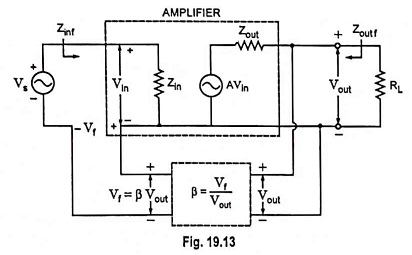The input impedance can be determined as follows: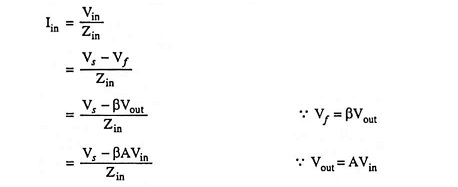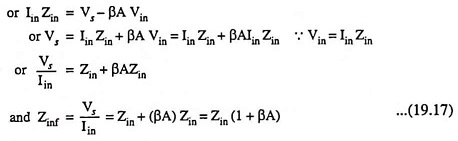The input impedance with series feedback is seen to have the value of input impedance without feedback multiplied by the factor (1 + βA) and applies to both voltage-series and current series configuration.

### Voltage-Shunt Feedback:

A more detailed shunt feedback connection is depicted in Fig. 19.14.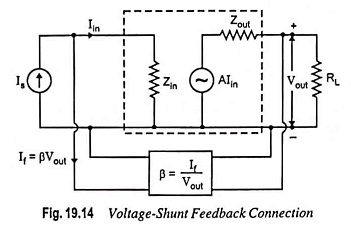In this case,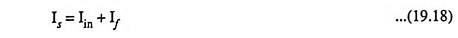and input impedance,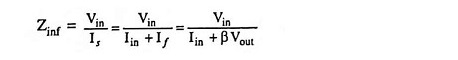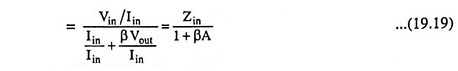So, the input impedance with voltage shunt feedback is reduced by the factor (1 + βA).

### Current-Shunt Feedback:

From Fig. 19.10(d)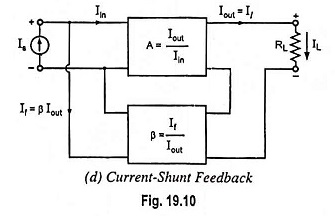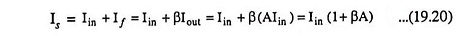and input impedance,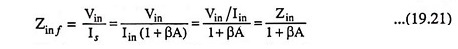So, the input impedance with current shunt feedback is reduced by the factor (1 + βA).

Scroll to Top